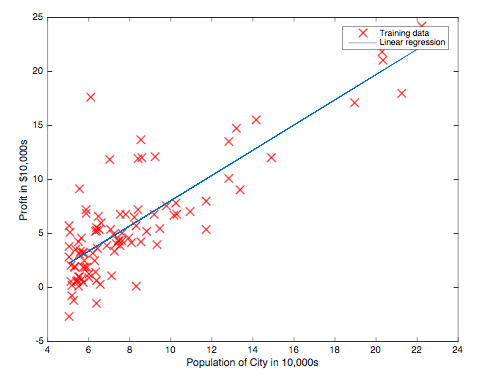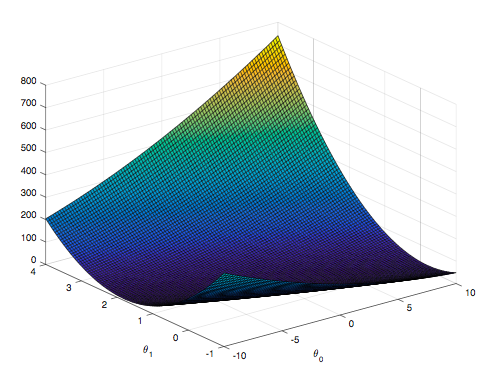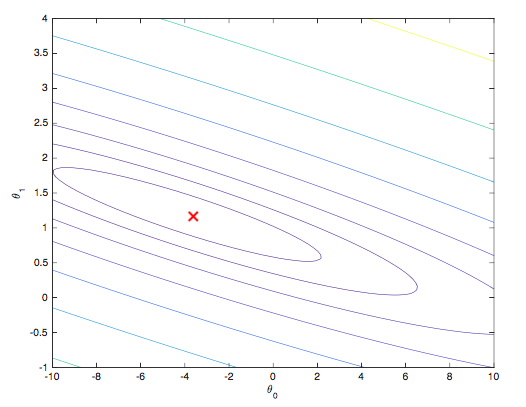In this part of this exercise from Coursera Machine Learning, I will implement linear regression with one variable to predict profits for a food truck. Matlab is the tools I used for this project. Link to Github

### Plotting the data

The first figure shows the scatter plot of profit and population in the city.The scatter plot of the training data reveals the positive relationship between profit and population. Also, the blue line is the regression line.

function plotData(x, y)

plot(x, y, 'rx', 'MarkerSize', 10); % Plot the data
ylabel('Profit in \$10,000s'); % Set the y?axis label
xlabel('Population of City in 10,000s'); % Set the x?axis label

figure; % open a new figure window

end


In this part, I will fit the linear regression parameters θ to our dataset using gradient descent. The objective of linear regression is to minimize the cost function

J = sum((X * theta - y) .^ 2) / (2*m);


where the hypothesis h0(x) is given by the linear model

For population = 35,000, we predict a profit of 4519.767868
For population = 70,000, we predict a profit of 45342.450129

% Predict values for population sizes of 35,000 and 70,000
predict1 = [1, 3.5] *theta;
predict2 = [1, 7] * theta;


function [theta, J_history] = gradientDescent(X, y, theta, alpha, num_iters)

m = length(y); % number of training examples
J_history = zeros(num_iters, 1);

for iter = 1:num_iters
theta = theta - (alpha/m) * (X' * (X * theta - y));
J_history(iter) = computeCost(X, y, theta);
end

end


#### Surface plot#### Contour plotfprintf('Visualizing J(theta_0, theta_1) ...\n')

% Grid over which we will calculate J
theta0_vals = linspace(-10, 10, 100);
theta1_vals = linspace(-1, 4, 100);

% initialize J_vals to a matrix of 0's
J_vals = zeros(length(theta0_vals), length(theta1_vals));

% Fill out J_vals
for i = 1:length(theta0_vals)
for j = 1:length(theta1_vals)
t = [theta0_vals(i); theta1_vals(j)];
J_vals(i,j) = computeCost(X, y, t);
end
end

% Because of the way meshgrids work in the surf command, we need to
% transpose J_vals before calling surf, or else the axes will be flipped
J_vals = J_vals';
% Surface plot
figure;
surf(theta0_vals, theta1_vals, J_vals)
xlabel('\theta_0'); ylabel('\theta_1');

% Contour plot
figure;
% Plot J_vals as 15 contours spaced logarithmically between 0.01 and 100
contour(theta0_vals, theta1_vals, J_vals, logspace(-2, 3, 20))
xlabel('\theta_0'); ylabel('\theta_1');
hold on;
plot(theta(1), theta(2), 'rx', 'MarkerSize', 10, 'LineWidth', 2);


Full source code: Github